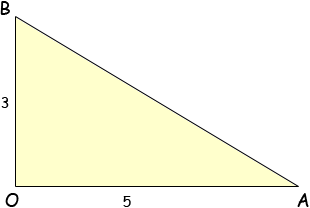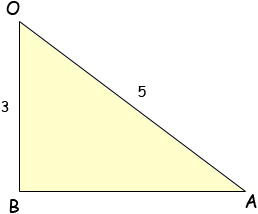Subject: GEOMETRY AND MENSURATION Name: SIDDHARTHA Who are you: Student area of a right angled triangle OAB where OA = 5 and OB = 3 Siddhartha, It depends where the right angle is. If the right angle is at O then you can situate the triangle this waywith a base length of 5 units and a height of 3 units. The area is then 1/2baseheight . If the right angle is at B then you can draw the triangle this wayand use Pythagoras theorem to find the length of the base BA before applying the area formula. Can the right angle be at A? If so what do you do? Penny